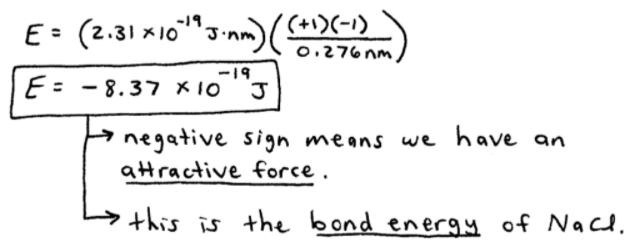# S8E1 - Coulomb's Law Examples and Potential Energy Diagrams

Everything around us is comprised of a complex mixture of chemical compounds.

And all of these compounds are bonded together with different kinds of atoms.

The manner in which atoms bind together has a profound effect on chemical and physical properties.

## Types of Chemical Bonds

Broadly speaking, there are two types of chemical bonds in Chemistry.

1. Ionic Bonds

2. Covalent Bonds

We'll talk a TON about covalent bonds in later posts, but for now let's focus on #1 - Ionic Bonds...

==========

## Ionic Bonds

Ionic Bonds  =  Ionic bonds are stronger than covalent bonds and have a high bond energy.

Bond energy  =  the energy (J) required to break a bond.

In other words, the stronger the bond, the more (bond) energy that's required to break it.

Remember...

Ionic compounds form when an atom that loses electrons easily (cation - usually a metal) reacts with an atom that gains electrons easily (anion - usually a nonmetal).

Bond energy is often referred to as the "energy of interaction" between cations and anions.

Here's a bond energy example, which uses Coulomb's Law...

----------

## Coulomb's Law Example

ex:  Find the energy of interaction ("the bond energy") between a pair of Na+ and Cl- ions, when the distance between them is 2.76Å (0.276nm).
_________

➞  to do this, we must use Coulomb's Law:

## Coulomb's Law Formula

E = (2.31 x 10-19 J.nm)(Q1Q2 / r)

➞  here's what I mean, visually:With Coulomb's Law, we can use the +1 charge on Na+, the -1 charge on Cl-, and the distance between the ion centers (0.276 nm), and plug them in to get:As stated in the image above, the negative sign means we have an attractive force between Na+ and Cl-.

This attractive force is the bond energy of NaCl.

==========

## Bond Length and Bond Energy

Bond Length  =  the distance between two atoms (or ions) where the energy is minimal.

See the plot that follows below.

Okay, so...

Bond Length vs. Bond Energy?

How are these two ideas related?

➞  the shorter the bond length, the stronger the bond,

➞  and therefore the harder it is to break the bond (higher bond energy).

----------

ex:  Consider the Potential Energy Diagram of H (H—H is a covalent bond).3 KEY POINTS:

1.  In the upper left, the 2 H's are too close together.

The one nucleus nucleus repulsion is severe.

It outweighs the two nucleus ⟷ electron attractions.

----------

2.  In the upper right, the 2 H's are so far apart that they can't "see" each other.

No interation.  (0 kJ/mol).

----------

3.  At the bottom of the plot, the H—H bond is the optimum length to achieve lower overall energy of a system.

The two nucleus ⟷ electron attractive interactions outweigh the nucleus ⟷ nucleus repulsion.

So...

==========

## What Determines if a Bond is Ionic or Covalent?

To answer this, you must consider the two atoms' or ions' differences in electronegativity.

Electronegativity  =  the ability of an atom to attract electrons to itself.

We'll talk all about electronegativity in my next video blog post...

==========

In my 2nd post in this series, SECTION 8 - Chemical Bonding, we'll discuss the video notes for